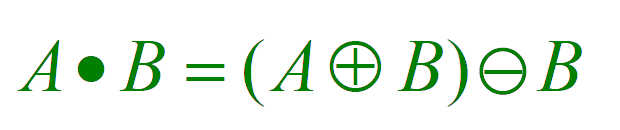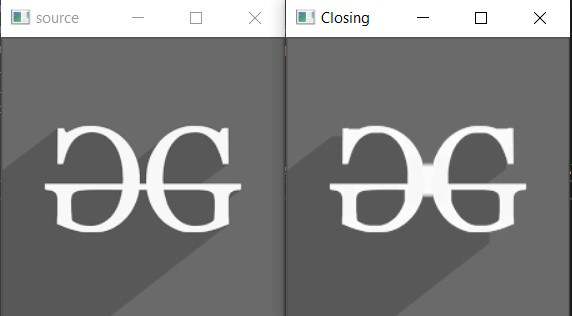# Closing | Morphological Transformations in OpenCV in C++

• Last Updated : 10 Feb, 2021

• It helps to reduce the internal noise present inside an image.
• In this article, another operator is elaborated called closing which is just the reverse of Opening and applies dilation followed by erosion.
• Just like the Opening operator it also uses a structuring element but it is used for removing small holes instead of pertusions.

Syntax:

morphologyEx (src, dst, op, kernel, anchor, iterations, borderType, borderValue)

Parameters:

• src: It is the input image.
• dst: It is the output image.
• op: Type of morphological operation.
• kernel: Structuring element used for Closing.
• anchor: Anchor position inside the structuring element. The default value is [-1, -1} signifying position as the center of the structuring element.
• iterations: Number of times Closing is applied.
• borderType: Type of border ( BORDER_CONSTANT, BORDER_REPLICATE, etc.)
• borderValue: Border value
• Return: Output Image (Mat Object)

The closing operation is given by the expression:• The expression represents the fact that A is a sub-image of A.B.
• This operator is used to remove small holes from the image.
• It also helps in smoothening the contours and fusion of narrow breaks and long thin gulfs.

Below is the program demonstrating the Closing Morphological Operator:

## C++

 `// C++ program for demonstrating the ` `// closing morphological operator ` ` `  `// For drawing shapes ` `#include ` `#include ` `using` `namespace` `cv; ` `using` `namespace` `std; ` ` `  `// Function to demonstrate the ` `// closing morphological operator ` `void` `closingMorphological() ` `{ ` `    ``// Reading the Image ` `    ``Mat image = imread( ` `        ``"C:/Users/harsh/Downloads/geeks.png"``, ` `        ``IMREAD_GRAYSCALE); ` ` `  `    ``// Check if the image is ` `    ``// created successfully ` `    ``if` `(!image.data) { ` `        ``cout << ``"Could not open or "` `             ``<< ``"find the image\n"``; ` `        ``return` `0; ` `    ``} ` ` `  `    ``int` `morph_size = 2; ` ` `  `    ``// Create structuring element ` `    ``Mat element = getStructuringElement( ` `        ``MORPH_RECT, ` `        ``Size(2 * morph_size + 1, ` `             ``2 * morph_size + 1), ` `        ``Point(morph_size, morph_size)); ` `    ``Mat output; ` ` `  `    ``// Closing ` `    ``morphologyEx(image, output, ` `                 ``MORPH_CLOSE, element, ` `                 ``Point(-1, -1), 2); ` ` `  `    ``// Displays the source and ` `    ``// the closing image formed ` `    ``imshow(``"source"``, image); ` `    ``imshow(``"Closing"``, output); ` `    ``waitKey(); ` `} ` ` `  `// Driver Code ` `int` `main(``int` `argc, ``char``** argv) ` `{ ` `    ``// Function Call ` `    ``closingMorphological(); ` ` `  `    ``return` `0; ` `} `

Output:My Personal Notes arrow_drop_up
Recommended Articles
Page :